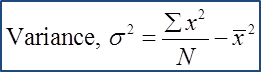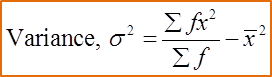# 7.3 Variance and Standard Deviation

Measures of Dispersion (Part 3)
7.3 Variance and Standard Deviation

1. The variance is a measure of the mean for the square of the deviations from the mean.

2. The standard deviation refers to the square root for the variance.

(A) Ungrouped DataExample 1:
Find the variance and standard deviation of the following data.
15, 17, 21, 24 and 31

Solution:

(B) Grouped Data (without Class Interval)Example 2:
The data below shows the numbers of children of 30 families:

 Number of child 2 3 4 5 6 7 8 Frequency 6 8 5 3 3 3 2

Find the variance and standard deviation of the data.

Solution:

(C) Grouped Data (with Class Interval)Example 3:

 Daily Salary(RM) Number of workers 10 – 14 40 15 – 19 25 20 – 24 15 25 – 29 12 30 – 34 8
Find the mean of daily salary and its standard deviation.

Solution:

 Daily Salary (RM) Number of workers, f Midpoint, x fx fx2 10 – 14 40 12 480 5760 15 – 19 25 17 425 7225 20 – 24 15 22 330 7260 25 – 29 12 27 324 8748 30 – 34 8 32 256 8192 Total 100 1815 37185